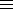### Solving the congruence ax2+bx+c=0 (mod n), gcd(a,n)=1

First solve t2b2-4ac (mod 4n), -n < t ≤ n.
Note that tb (mod 2).
Then solve ax(t-b)/2 (mod n), 0 ≤ x < n.

Enter a (nonzero):
Enter b:
Enter c:
Enter n (≥ 1 and gcd(a,n)=1):